Name: ___________________Date:___________________

 Email us to get an instant 20% discount on highly effective K-12 Math & English kwizNET Programs!

### Grade 4 - Mathematics8.9 Types of Fractions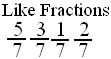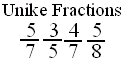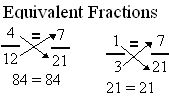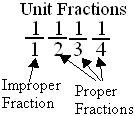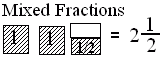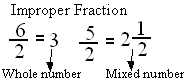Directions: Answer the following questions. Also write at least 5 examples of each type of fractions.
 Q 1: A fraction less than 1 is called a proper fraction. A fraction equal to one and more than 1 is called _________.mixed fractionimproper fractionproper fraction Q 2: Fractions with different denominators are calledunlike fractionslike fractions Q 3: If the numerator is less than the denominator in a fraction, the fraction is called a _______.mixed fractionproper fractionimproper fraction Q 4: A number with a whole number and a proper fraction is aproper numbermixed number Question 5: This question is available to subscribers only! Question 6: This question is available to subscribers only!